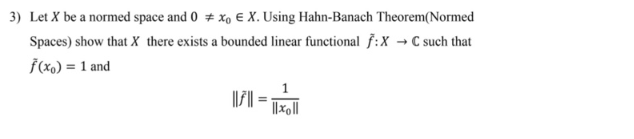## Solve using Hahn-Banach Theorem.Transcript:
3) Let X be a normed space and 0 # xo e X. Using Hahn-Banach Theorem(Normed Spaces) show that X there exists a bounded linear functional f:X + C such that f(x) = 1 and 1 ||||| = ||xg||

Solved
Applied Mathematics 1 Answer Anonymous(s) Post